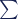# Variance, Standard Deviation and Standard Error: Maths and Stats

### Fast facts

• Both variance and standard deviation are measures of spread.
• Standard deviation is equal to the square root of the variance.
• Standard deviation is used to describe the data, and standard error is used to describe statistical accuracy.
• It is easier to calculate these using software than by hand.

### Variance

Variance is a measure of how far the observed values in a dataset fall from the arithmetic mean, and is therefore a measure of spread - more specifically, it is a measure of variability. It is denoted by the Greek letter sigma squared, and its formula is given by:where:

• σ2 is the variance we are wanting to find
•is the summation function
• x is an observation in the dataset
•is the population mean
• n is the number of observations in the population.

### Standard Deviation

Standard deviation is the square root of the variance, and therefore is also a measure of spread - more specifically, it is a measure of dispersion (or, the measure of variability!). Where variance is used to show how much the values in a dataset vary from each other, the standard deviation exists to show how far apart the values in a dataset are from the mean, and therefore can be used to identify outliers.

Standard deviation is denoted by the Greek letter sigma and, being the square root of variance, is written as:where:

• σ2 is the variance we are wanting to find
•is the summation function
• x is an observation in the dataset
•is the population mean
• n is the number of observations in the population.

### Standard Error

Standard error is another measure of spread. The most common standard error is the standard error of the mean, and used to measure sampling error as it measures how accurately the mean of a sample distribution represents the mean of the population. In other words, it shows how much variation there is likely to be between different samples of a population and the population itself.

The main difference between the standard deviation and the standard error is that the standard deviation is a type of descriptive statistics, used to summarise the data, whereas the standard error of the mean describes the random sampling process, and is an estimation rather than a definite value like the standard deviation is. It is useful because you can see how well your sample data represents your population.

The formula is given by:where:

• SE is the standard error
• σ is the standard deviation
• is the sample size.

### Examples

#### Example 1

Let's say we have the following dataset:

7, 12, 5, 18, 5, 9, 10, 9, 12, 8, 12, 16

In order to find the variance and standard deviation of this, we need to first find the mean, which is:The variance of this dataset is then given by:to two decimal places.

Then, the standard deviation is:to two decimal places, and the standard error is given by:to two decimal places.

#### Example 2

Calculating the variance and standard deviation by hand is a long-winded process, and with large datasets there is much room for human error. Using software for these sorts of calculations tends to be the more ideal thing to do.

• To calculate the variance and standard deviation of the above dataset in R, we can create a variable for the data and then calculate the variance and standard deviation with the var and sd functions respectively:

dataset <- c(7, 12, 5, 18, 5, 9, 10, 9, 12, 8, 12, 16)

var(dataset)

sd(dataset)

To find the standard error, you can define your own function to be simply the standard deviation divided by the square root of n and apply that function to the dataset:

standard.error <- function(x) sd(x)/sqrt(length(x))

standard.error(dataset)

• To calculate the variance and standard deviation in Excel, you can use the VAR.S and STDEV functions respectively since the dataset is all numeric. If the dataset is contained in the cells A1 to A12, then the function you would write would be:

=VAR.S(A1:A12)

=STDEV(A1:A12)

The standard error will need to be written out yourself, using the above STDEV function with the COUNT function to find n:

=STDEV(A1:A12)/SQRT(COUNT(A1:A12))